# Introduction

This package contains a C++ implementation of the RecMap algorithm ,  to draw maps according to given statistical values. These so-called cartograms or value-by-area-maps may be used to visualize any geospatial-related data, e.g., political, economic or public health data. The input consists of a map represented by overlapping rectangles. This map is defined by the following parameters for each map region:

• a tuple of (x, y) values corresponding to the (longitude, latitude) position,
• a tuple of (dx, dy) of expansion along (longitude, latitude),
• and a statistical value z.

The (x, y) coordinates represent the center of the minimal bounding boxes (MBB), The coordinates of the MBB are derived by adding or subtracting the (dx, dy) tuple accordingly. The tuple (dx, dy) also defines the ratio of the map region. The statistical values define the desired area of each map region.

The output is a rectangular cartogram where the map regions are:

• Non-overlapping,
• connected,
• ratio and area of each rectangle correspond to the desired areas,
• rectangles are placed parallel to the axes.

The construction heuristic places the rectangles in a way that important spatial constraints, in particular

• the topology of the pseudo dual graph,
• the relative position of MBB centers.

are tried to be preserved.

The ratios are preserved, and the area of each region corresponds to the as input given statistical value z.

The graphic below depicts a typical example of a rectangular cartogram drawing.

Rectangular Cartogram of the U.S. election 2004; The area corresponds to the number of electors (color indicates the party red: democrats / blue: Republican; the color intensity ~ outcome of the vote.). The graphic was computed by using the original implementation of the construction heuristic RecMap MP2 introduced in .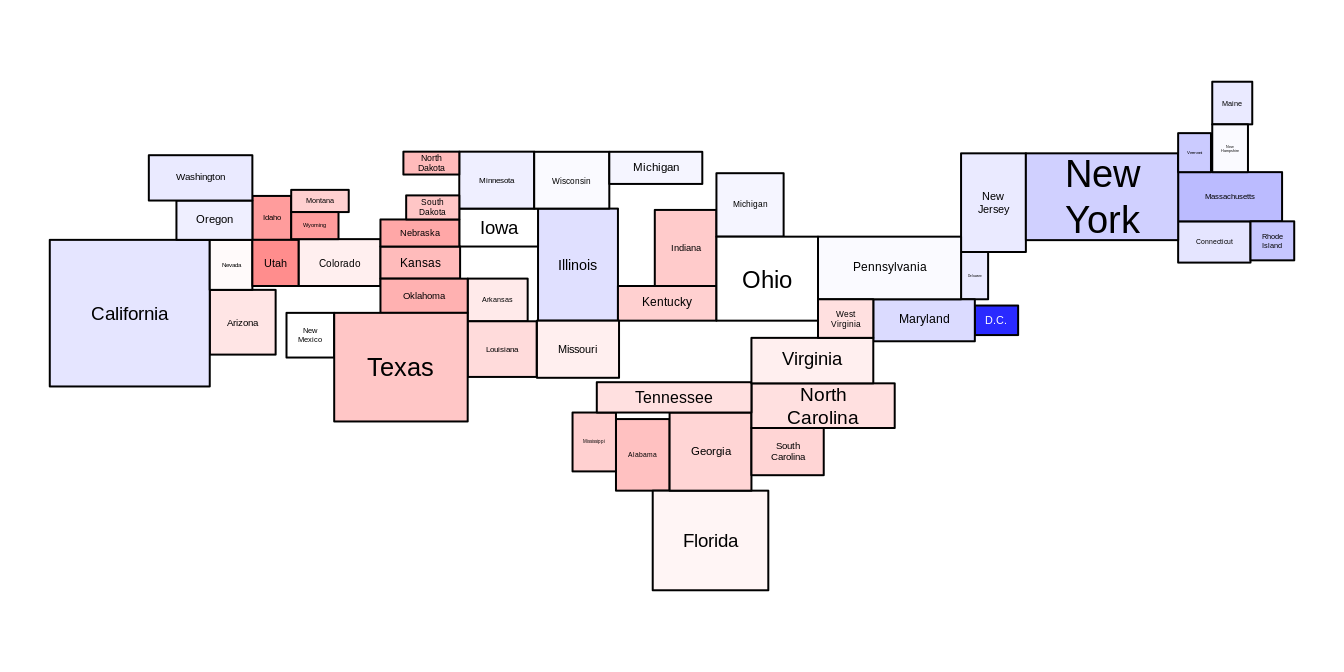# The Usage

attach the package

library(recmap)

look into for documentation

help(package="recmap") 

## Input - using the U.S. state Facts and Figures Dataset

usa <- data.frame(x = state.center$x, y = state.center$y,
# make the rectangles overlapping by correcting lines of longitude distance
dx = sqrt(state.area) / 2 / (0.8 * 60 * cos(state.center$y*pi/180)), dy = sqrt(state.area) / 2 / (0.8 * 60) , z = sqrt(state.area), name = state.name) ## Compute Pseudo Dual Graph (PD) The rectangles have to overlap to compute the dual graph. This enables to generate valid input having only the (x, y) coordinates of the map region. library(recmap) op <- par(mfrow = c(1 ,1), mar = c(0, 0, 0, 0), bg = NA) plot.recmap(M <- usa[!usa$name %in% c("Hawaii", "Alaska"), ],
col.text = 'black', lwd=2)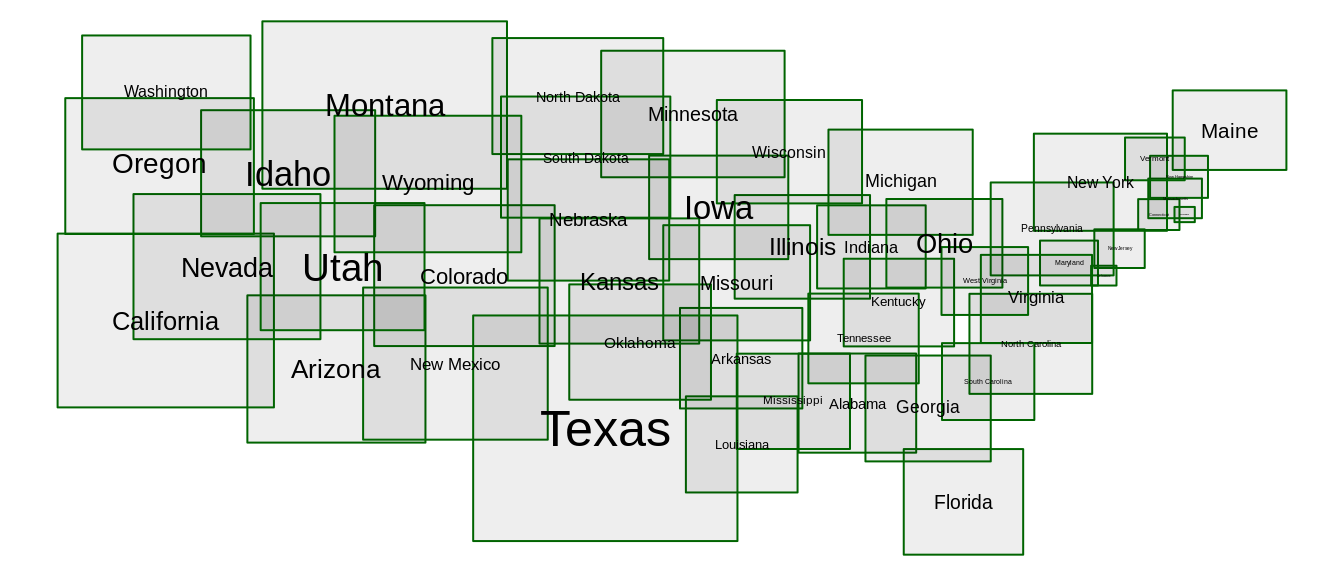## Apply a Metaheuristic

The index order of the input map has an impact to the resulting cartogram. This algorithmic property is caused due to the computation of the dual graph. In  a genetic algorithm was applied as metaheuristic. Due to the limited computing resources on the CRAN check systems, we do not use all the potential of the metaheuristic.

Study the examples of the reference manual ?recmapGA on how the GA package can be used.

## Objective Functions

The topology error is an indicator of the deviation of the neighborhood relationships. The error is computed by counting the differences between dual graphs or adjacency graphs of map and cartogram

The relative positions error measures the angle difference between all region centers.

## Output

The output is a data.frame object.

Cartogram <- recmap(Map <- usa[!usa$name %in% c("Hawaii", "Alaska"), ]) head(Cartogram) ## x y dx dy z name dfs.num ## 1 -86.12445 24.40204 3.201992 2.697824 227.17614 Alabama 31 ## 2 -123.13242 29.61981 3.939586 3.257613 337.50407 Arizona 38 ## 3 -85.30009 39.65352 3.265364 2.683509 230.44305 Arkansas 33 ## 4 -131.42392 31.53933 4.341915 3.488740 398.36290 California 37 ## 5 -122.00152 23.21422 3.965671 3.095895 322.87304 Colorado 47 ## 6 -53.13517 41.31657 1.896954 1.418697 70.77429 Connecticut 19 ## topology.error relpos.error relposnh.error ## 1 4 0.2265446 0.5912115 ## 2 4 0.2041032 0.7446224 ## 3 6 0.4400093 0.8416664 ## 4 3 0.1353180 0.1454522 ## 5 8 0.5045327 0.9388417 ## 6 3 0.1937878 0.4808290 # Application ## Rectangular Map Approximation smp <- c(29, 22, 30, 3, 17, 8, 9, 41, 18, 15, 38, 35, 21, 23, 19, 6, 31, 32, 20, 28, 48, 4, 13, 14, 42, 37, 5, 16, 36 , 43, 25, 33, 12, 7, 39, 44, 2, 47, 45, 46, 24, 10, 1,11 ,40 ,26 ,27 ,34) op <- par(mfrow = c(1 ,1), mar = c(0, 0, 0, 0), bg = NA) plot(Cartogram.Area <- recmap(M[smp, ]), col.text = 'black', lwd = 2) Rectangular Map Approximation - rectangle area correspond to state area.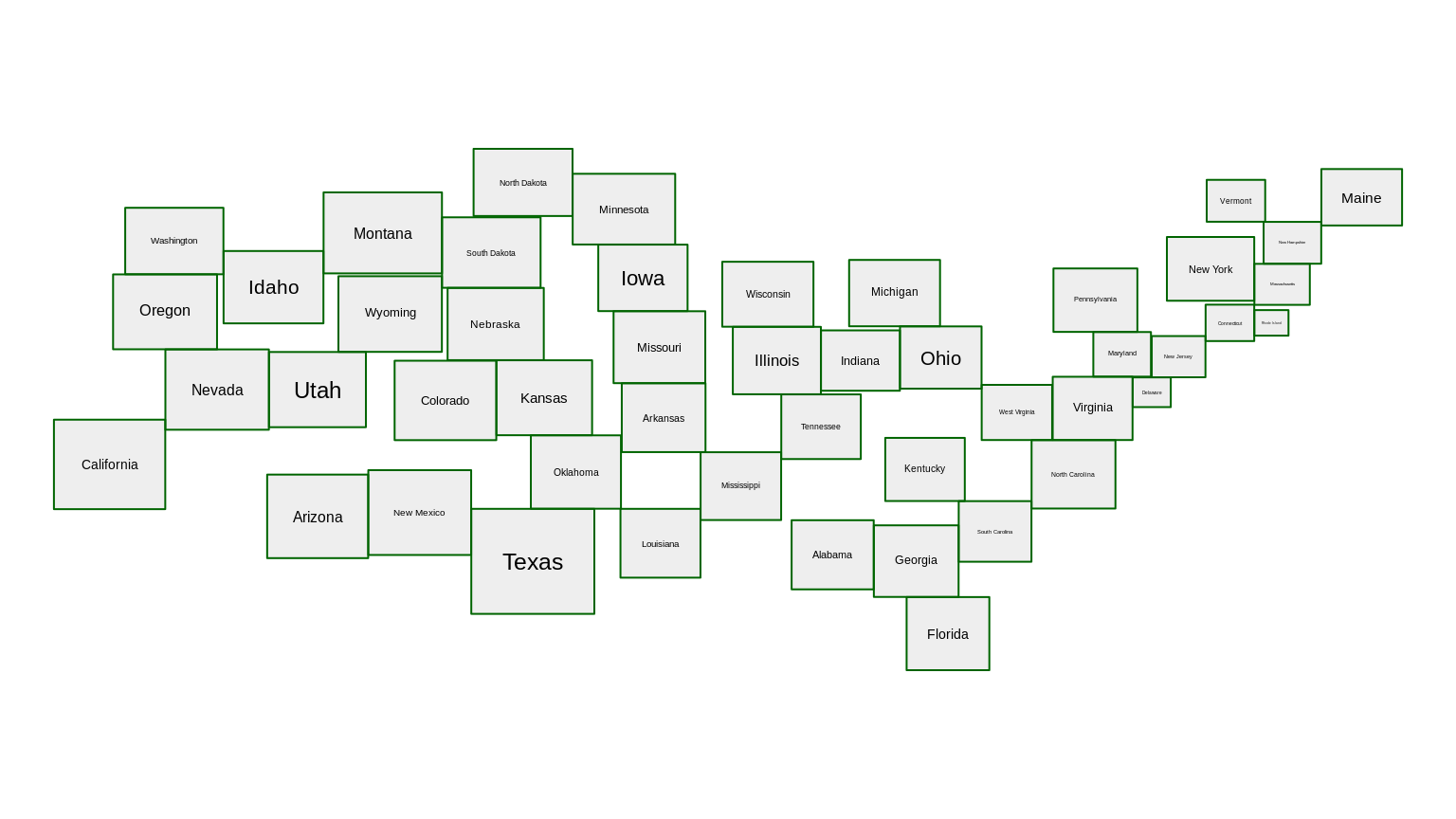summary.recmap(M) ## values ## number of map regions 48.00000 ## area error 0.13000 ## topology error NA ## relative position error NA ## screen filling [in %] 113.77072 ## xmin -124.93741 ## xmax -66.26565 ## ymin 25.35365 ## ymax 50.81868 summary(Cartogram.Area) ## values ## number of map regions 48.00000 ## area error 0.00000 ## topology error 156.00000 ## relative position error 0.09000 ## screen filling [in %] 39.74965 ## xmin -131.88541 ## xmax -26.73219 ## ymin 17.21867 ## ymax 57.88620 ## state.x77[, 'Population'] op <- par(mfrow = c(1 ,1), mar = c(0, 0, 0, 0), bg = NA) usa$z <- state.x77[, 'Population']
M <- usa[!usa$name %in% c("Hawaii", "Alaska"), ] plot(Cartogram.Population <- recmap(M[order(M$x), ]),
col.text = 'black', lwd = 2)

Area ~ population estimate as of July 1, 1975;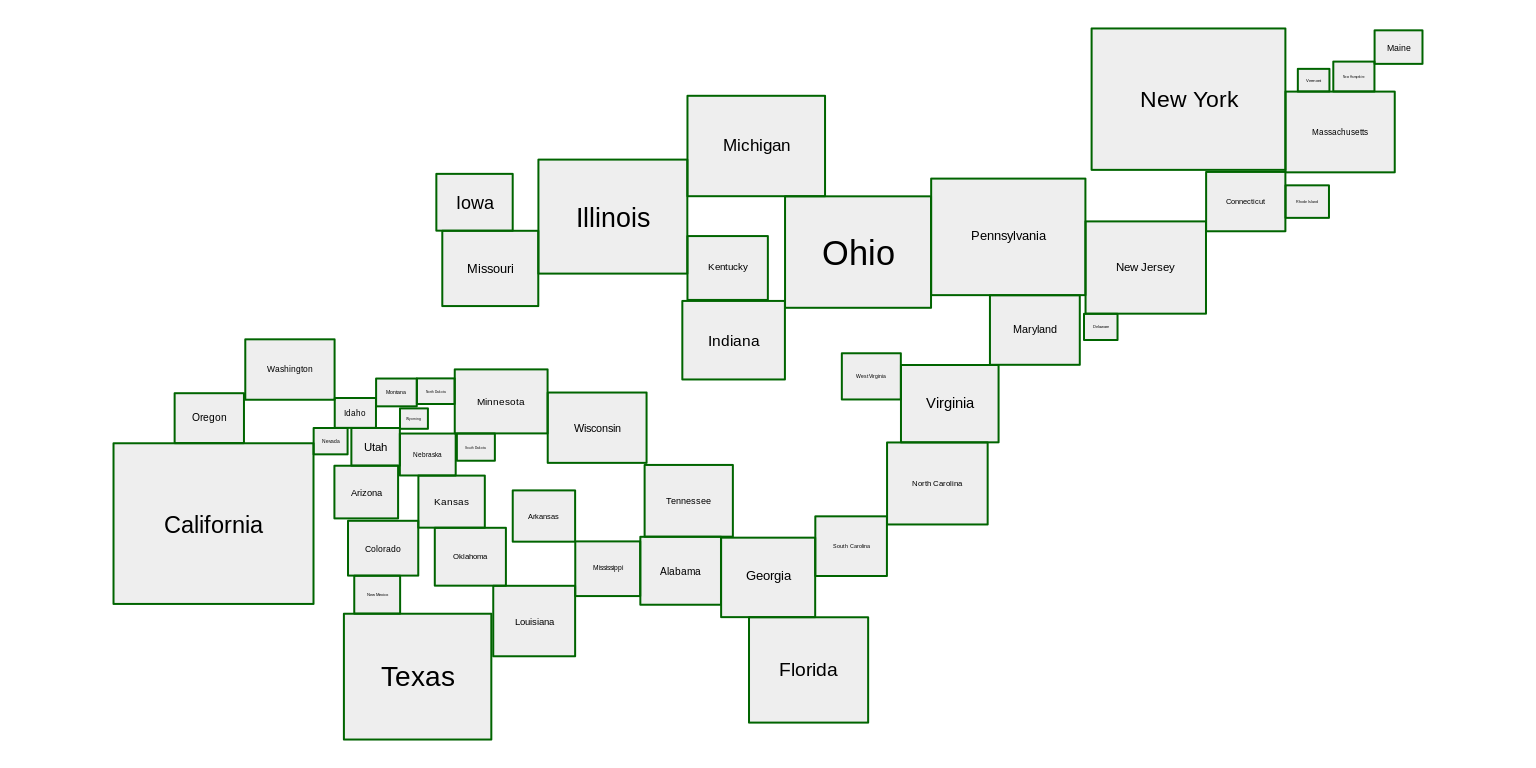# index order

smp <- c(20,47,4,40,9,6,32,33,3,10,34,22,2,28,15,12,39,7,42,45,19,13,43,30,24,
25,11,17,37,41,26,29,21,35,8,36,14,16,31,48,46,38,23,18,1,5,44,27)

op <- par(mfrow = c(1 ,1), mar = c(0, 0, 0, 0), bg = NA)
plot(Cartogram.Population <- recmap(M[smp,]), col.text = 'black', lwd = 2)

Area ~ population estimate as of July 1, 1975; a better index order has been chosen to minimize the relative position error.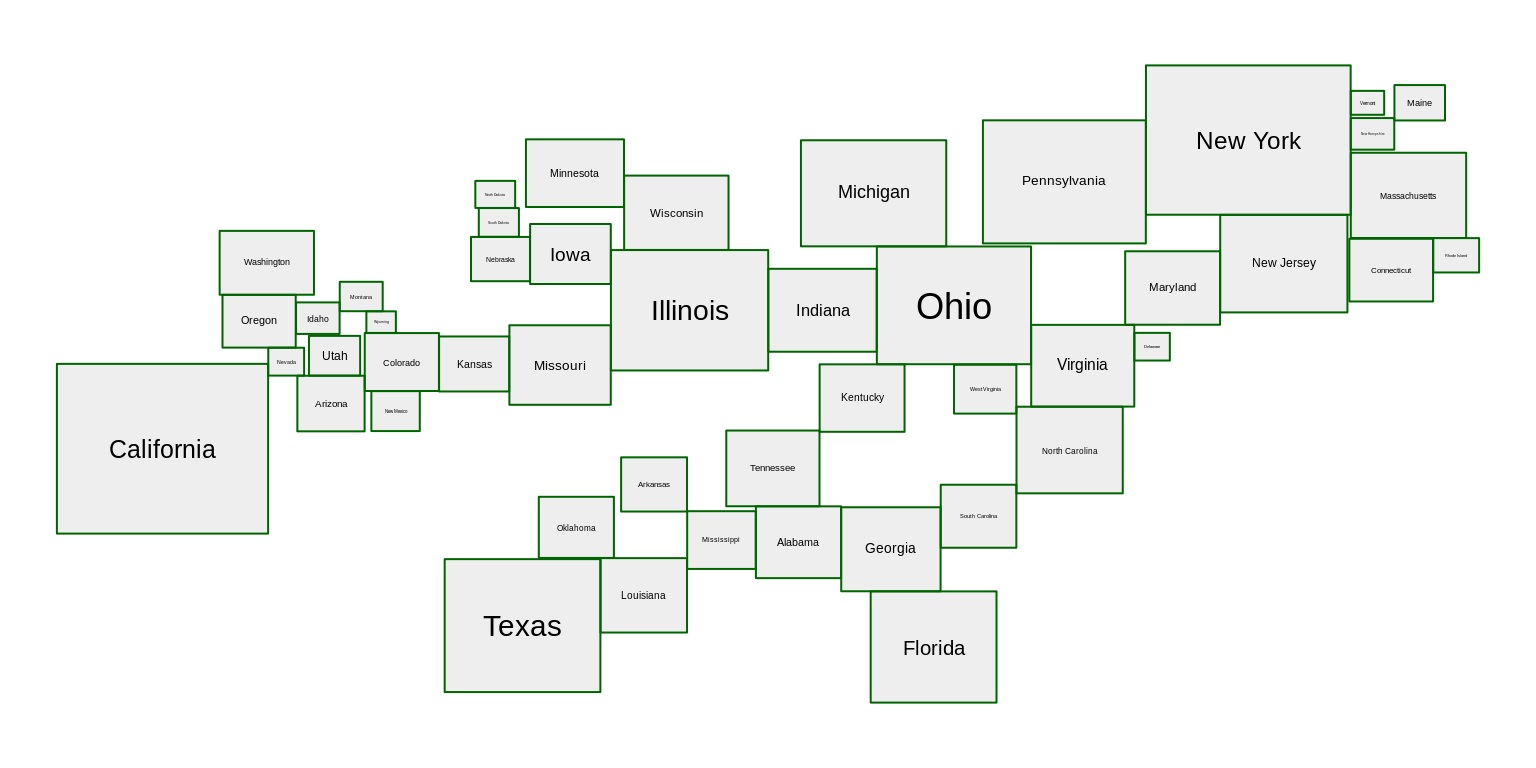## state.x77[, 'Income']

usa$z <- state.x77[, 'Income'] M <- usa[!usa$name %in% c("Hawaii", "Alaska"), ]
op <- par(mfrow = c(1 ,1), mar = c(0, 0, 0, 0), bg = NA)
plot(Cartogram.Income <- recmap(M[order(M$x),]), col.text = 'black', lwd = 2) Area ~ capita income (1974);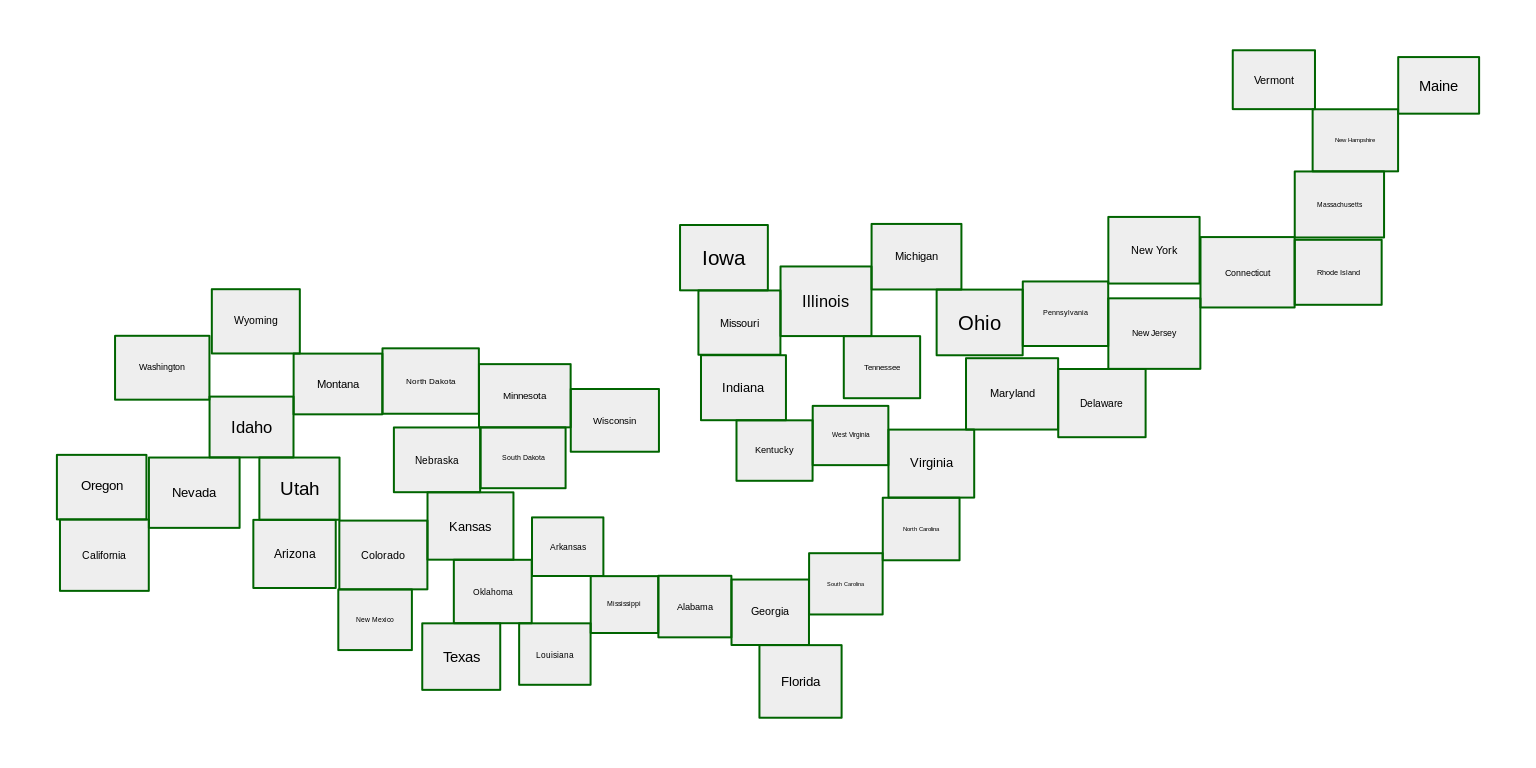## state.x77[, 'Frost'] usa$z <- state.x77[, 'Frost']
M <- usa[!usa$name %in% c("Hawaii", "Alaska"), ] op <- par(mfrow = c(1 ,1), mar = c(0, 0, 0, 0), bg = NA) Frost <- recmapGA(M, seed = 1) plot(Frost$Cartogram,
col.text = 'black', lwd = 2)

Area ~ mean number of days with minimum temperature below freezing (1931<U+2013>1960) in capital or large city;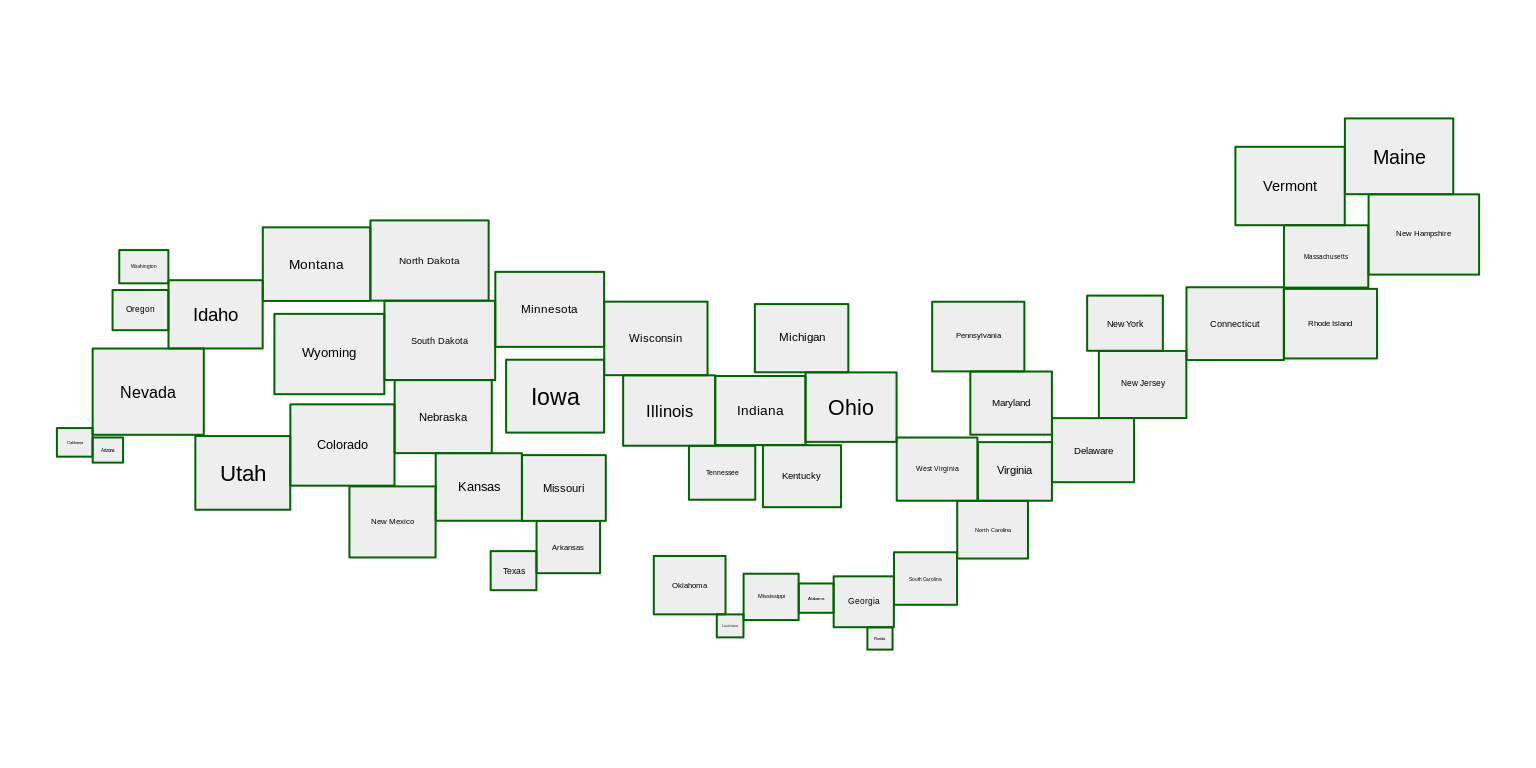summary(Frost)
## summary of class recmapGA:
## summary of the GA:
## -- Genetic Algorithm -------------------
##
## GA settings:
## Type                  =  permutation
## Population size       =  480
## Number of generations =  10
## Elitism               =  24
## Crossover probability =  0.8
## Mutation probability  =  0.25
##
## GA results:
## Iterations             = 10
## Fitness function value = 0.1572351
## Solution =
##      x1 x2 x3 x4 x5 x6 x7 x8 x9 x10  ...  x47 x48
## [1,] 30  3 27 19 20 15 28 17 40  41        25  43
##                                Map  Cartogram
## number of map regions     48.00000   48.00000
## area error                 0.30000    0.00000
## topology error                  NA  156.00000
## relative position error         NA    0.13000
## screen filling [in %]    113.77072   34.24012
## xmin                    -124.93741 -133.14775
## xmax                     -66.26565  -17.85919
## ymin                      25.35365   24.12804
## ymax                      50.81868   67.18886

More interactive examples using state.x77 data are available by running the code snippet below.

# Requires to install the suggested packages
# install.packages(c('colorspace', 'maps', 'noncensus', 'shiny'))

library(shiny)

recmap_shiny <- system.file("shiny-examples", package = "recmap")
shiny::runApp(recmap_shiny, display.mode = "normal")

## Synthetic input maps - checkerboard

Checkerboards provide examples of sets of map regions which do not have ideal cartogram solutions according to Definition 1 .

op <- par(mar = c(0, 0, 0, 0), mfrow = c(1, 3), bg = NA)

plot(checkerboard8x8 <- checkerboard(8),
col=c('white','white','white','black')[checkerboard8x8$z]) # found by a greedy randomized search index.greedy <- c(8, 56, 18, 5, 13, 57, 3, 37, 62, 58, 7, 16, 40, 59, 17, 34, 29, 41, 46, 27, 54, 43, 2, 21, 38, 52, 31, 20, 28, 48, 1, 22, 55, 11, 25, 19, 50, 10, 24, 53, 47, 30, 45, 44, 32, 35, 51, 15, 64, 12, 14, 39, 26, 6, 42, 33, 4, 36, 63, 49, 60, 61, 9, 23) plot(Cartogram.checkerboard8x8.greedy <- recmap(checkerboard8x8[index.greedy,]), col = c('white','white','white','black')[Cartogram.checkerboard8x8.greedy$z])

# found by a genetic algorithm
index.ga <- c(52, 10, 27, 63, 7, 20, 32, 18, 47, 28, 6, 55, 11, 61, 38, 50, 5,
21, 36, 34, 2, 22, 3, 1, 29, 57, 43, 4, 51, 58, 31, 49, 44, 25,
59, 33, 17, 40, 8, 41, 26, 37, 19, 56, 45, 35, 62, 53, 24, 64,
30, 15, 39, 12, 60, 48, 16, 23, 46, 42, 13, 54, 14, 9)

plot(Cartogram.checkerboard8x8.ga <- recmap(checkerboard8x8[index.ga,]),
col = c('white','white','white','black')[Cartogram.checkerboard8x8.ga\$z])

checkerboard fun - input, area of black regions have to be four times as big as white regions (left); solution found by a greedy random algorithm (middle); solution found by genetic algorithm (right)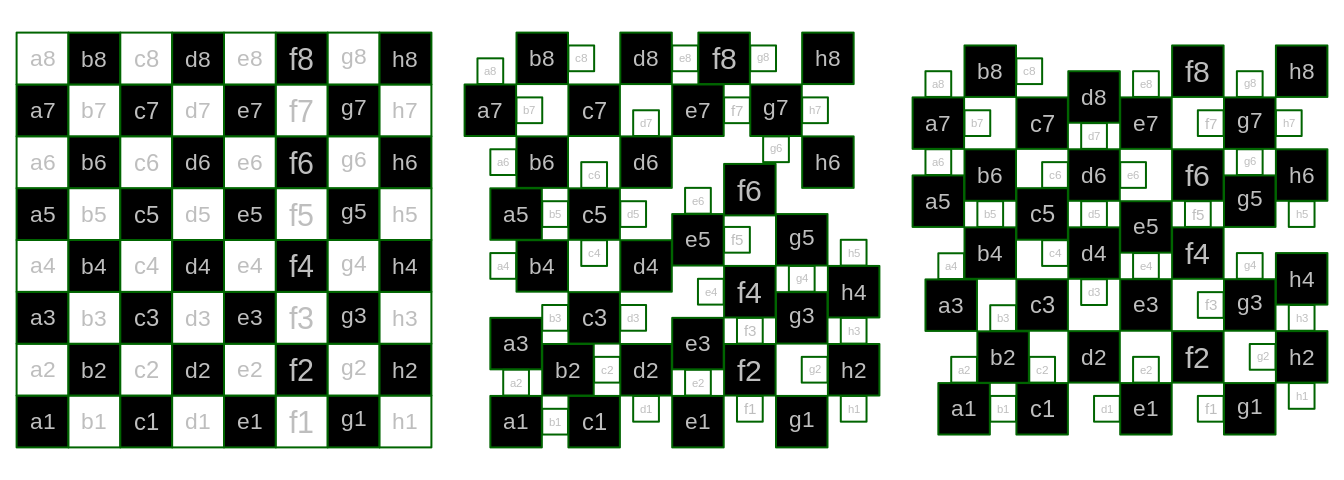# History

The work on RecMap was initiated by understanding the limits of contiguous cartogram drawing  and after studying the visualizations drawn by Erwin Raisz . The purpose of the first implementation  was a feasibility check on how computer-generated rectangular cartograms with zero area error could look like. The recmap R package on CRAN provides a rectangular cartogram algorithm to be used by any R user. Now, it is easy to generate input (e.g., no complex polygon mesh), the code is maintainable (less than 500 lines of C++-11 code), and the algorithm is made robust to the price of not having all features implemented (simplified local placement; no empty space error; no MP1 variant yet). Recent research publication on rectangular cartograms drawing include , , , . However, according to recent publication , recmap remains the only rectangular cartogram algorithm that ‘maintain zero cartographic error’.

# Session Info

sessionInfo()
## R version 4.0.3 (2020-10-10)
## Platform: x86_64-pc-linux-gnu (64-bit)
## Running under: Debian GNU/Linux 10 (buster)
##
## Matrix products: default
## BLAS:   /usr/lib/x86_64-linux-gnu/blas/libblas.so.3.8.0
## LAPACK: /usr/lib/x86_64-linux-gnu/lapack/liblapack.so.3.8.0
##
## locale:
##  C
##
## attached base packages:
##  stats     graphics  grDevices utils     datasets  methods
##  base
##
## other attached packages:
##  recmap_1.0.9     sp_1.4-4         Rcpp_1.0.5
##  GA_3.2           iterators_1.0.13 foreach_1.5.1
##
## loaded via a namespace (and not attached):
##   lattice_0.20-41  codetools_0.2-18 tufte_0.9
##   fansi_0.4.1      crayon_1.3.4     assertthat_0.2.1
##   digest_0.6.27    grid_4.0.3       magrittr_1.5
##  evaluate_0.14    highr_0.8        cli_2.1.0
##  rlang_0.4.8      stringi_1.5.3    rmarkdown_2.5
##  tools_4.0.3      stringr_1.4.0    glue_1.4.2
##  xfun_0.19        yaml_2.2.1       compiler_4.0.3
##  htmltools_0.5.0  knitr_1.30